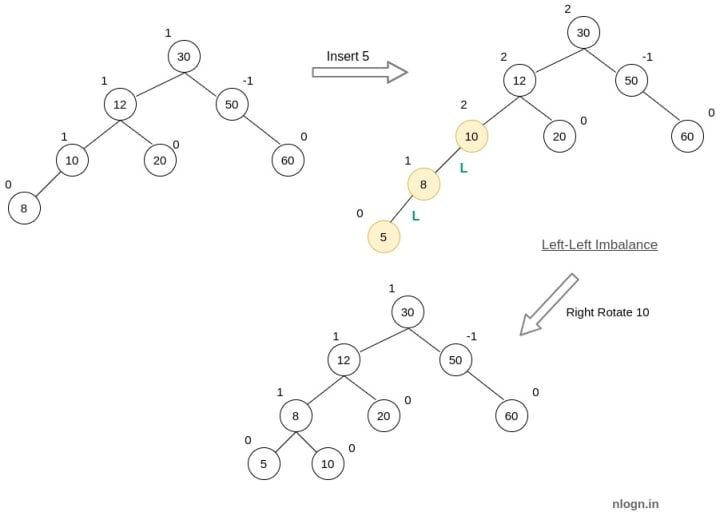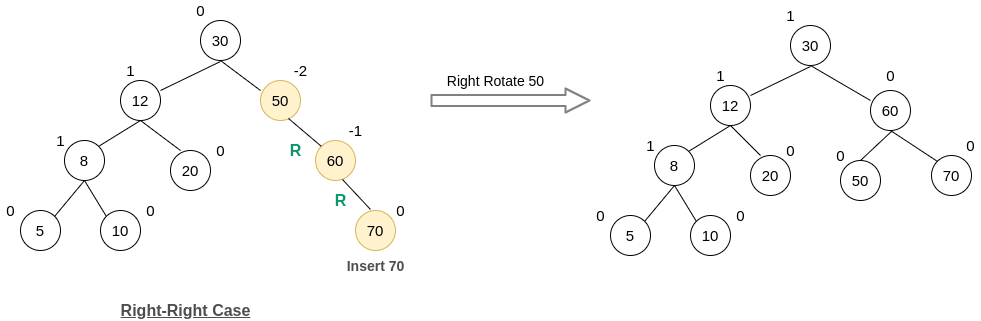Home Data Structures AVL Tree Insertion Tutorial

# AVL Tree Insertion Tutorial

AVL tree is a height-balanced Binary Search Tree(BST) with best and worst-case height as O(log N). In AVL tree the difference of height of left and right subtree (BalanceFactor) is always 1 or 0 or -1. Read more about AVL Tree and its properties here

### Insertion in AVL Tree

Insertion in AVL is similar to insertion in BST except after any insertion the tree should be balanced i.e, the BalanceFactor ∈ {1, 0, -1}. In case this property is violated we will do rotations to rebalance the tree.

##### Rotation in an AVL tree

In an AVL tree, we have to do Left or Right rotation for rebalancing a tree in case of an imbalance. Below is the representation of how the tree structure will change after a rotation.

```Let T1, T2 are subtrees of the tree rooted with x
and x & T3 are subtree of tree rooted with y

y                               x
/ \     Right Rotation          /  \
x   T3   - - - - - - - >        T1   y
/ \       < - - - - - - -            / \
T1  T2     Left Rotation            T2  T3
Keys in both of the above trees follow the
following order
keys(T1) < key(x) < keys(T2) < key(y) < keys(T3)
So BST property is not violated anywhere.```
##### Various cases in which rotations are done
###### 1. Left-Left case

In the following tree, x is left child of y and y is the left child of z, hence to balance this tree we have to right rotate this tree around the node z.

###### 2. Left-Right Case

In the following tree, x is the right child of y, and y is the left child of z, hence to balance this tree first we have to left rotate the tree around the node y and then right rotate the resulting tree around the node z.

###### 3. Right-Right Case

In the following tree, x is the right child of y, and y is the right child of z, hence to balance this tree we have to left rotate this tree around the node z.

###### 4. Right-Left Rotation

In the following tree, x is the left child of y, and y is the right child of z, hence to balance this tree first we have to right rotate the tree around the node y and then left rotate the resulting tree around the node z.

##### Steps for insertion

Suppose we are given an AVL tree T and N is the new node to be inserted.

1. Perform a standard BST insertion of node N in the AVL tree T.
2. Starting from node N, traverse up until the first unbalanced node is not found. Let z be the first unbalanced node, y be the child of z that comes on the path from p to z and x be the grandchild of z that comes on the path from p to z.
3. Rebalance the tree by performing suitable rotations on the subtree. There can be the following 4 possible cases and after choosing what case is it, we have to do appropriate rotation explained above.
1. Left-Left Case – y is left child of z and x is left child of y
2. Left-Right Case – y is left child of z and x is the right child of y
3. Right-Right Case – y is the right child of z and x is the right child of y
4. Right-Left Case – y is the right child of z and x is left child of y

Note: We only need to re-balance the subtree where the first imbalance has occurred using the above cased and the complete tree automatically becomes height-balanced.

##### Example

Let’s understand the steps of doing insertion using the following example. Given an AVL tree with nodes 30, 12, 50, 10, 20, 60, 8, insert the following nodes in a given AVL Tree – 5, 70, 90, 80

Insert 5Insert 70Insert 90 and 80##### Algorithm
```insert_AVL(tree, key){
//normal BST insertion
if(tree == NULL)
return new Node(value);
if(tree->value > key)
tree->left = insert_AVL(tree->left, key)
else if(tree->value > key)
tree->right = insert_AVL(tree->right, key)
else  //Duplicate keys are not allowed in BST
return tree;

left_height = height(tree->left);
right_height = height(tree->right);

//find height & balance factor of current node
tree->height = 1+max(left_height, right_height);
balance_factor = left_height - right_height;

//left-left case
if(balance_factor > 1 && key < tree->left->value){
return right_rotate(tree)
}

//left-right case
if(balance_factor > 1 && key > tree->left->value){
tree->left = left_rotate(tree->left)
return right_rotate(tree)
}

//right-right case
if(balance_factor < -1 && key > tree->left->value){
return right_rotate(tree)
}

//right-left case
if(balance_factor < -1 && key < tree->right->value){
tree->right = right_rotate(tree->right)
return left_rotate(tree)
}

return tree
}```
##### Time Complexity

The time complexity of insertion in an AVL tree is O(log N), where N, is the number of nodes in the tree. The important thing to remember is that the rotation operations (left and right rotate) take constant time O(1) as only a few pointers are being changed there. Updating the height and getting the balance factor also takes constant time.